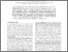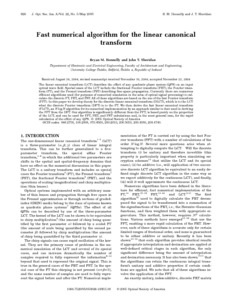# Fast numerical algorithm for the linear canonical transform

Hennelly, Bryan M. and Sheridan, John T. (2005) Fast numerical algorithm for the linear canonical transform. Journal of the Optical Society of America A., 22 (5). pp. 928-937. ISSN 1084-7529Previewmore...Add this article to your Mendeley library

## Abstract

The linear canonical transform (LCT) describes the effect of any quadratic phase system (QPS) on an input optical wave field. Special cases of the LCT include the fractional Fourier transform (FRT), the Fourier transform (FT), and the Fresnel transform (FST) describing free-space propagation. Currently there are numerous efficient algorithms used (for purposes of numerical simulation in the area of optical signal processing) to calculate the discrete FT, FRT, and FST. All of these algorithms are based on the use of the fast Fourier transform (FFT). In this paper we develop theory for the discrete linear canonical transform (DLCT), which is to the LCT what the discrete Fourier transform (DFT) is to the FT. We then derive the fast linear canonical transform (FLCT), an NlogN algorithm for its numerical implementation by an approach similar to that used in deriving the FFT from the DFT. Our algorithm is significantly different from the FFT, is based purely on the properties of the LCT, and can be used for FFT, FRT, and FST calculations and, in the most general case, for the rapid calculation of the effect of any QPS.

Item Type: Article Fast numerical algorithm; linear canonical transform; quadratic phase system (QPS); input optical wave field; fractional Fourier transform (FRT); Fourier transform (FT); Fresnel transform (FST); Faculty of Science and Engineering > Computer ScienceFaculty of Science and Engineering > Electronic EngineeringFaculty of Science and Engineering > Research Institutes > Hamilton Institute 8719 https://doi.org/10.1364/JOSAA.22.000928 Dr. Bryan Hennelly 04 Sep 2017 11:25 Journal of the Optical Society of America A. Optical Society of America Yes Enterprise Ireland, Science Foundation Ireland, Irish Research Council for Science Engineering and Technology (IRCSET)Item control page